# NCERT Exemplar Class 11 Chemistry Solutions for Chapter 13 - Hydrocarbons

## NCERT Exemplar Solutions Class 11 Chemistry Chapter 13 – Free PDF Download

NCERT Exemplar Solutions for Chemistry Class 11 Chapter 13 Hydrocarbons will guide students in understanding the topics from the exam point of view. They help students to grasp Hydrocarbon topics thoroughly in order to excel in the CBSE Class 11 final examination and graduate entrance examinations like IIT-JEE, JEE Advanced, AIEEE, AIPMT, NEET, etc. The NCERT Exemplar Class 11 Chemistry Chapter 13 PDF has answers to the questions given in the NCERT Exemplar book, important questions from previous years’ question papers, NEET questions, MCQs, short answer questions, exercises and worksheets.

After studying this NCERT Exemplar, you will be able to make important notes, which are crucial for the preparation for exams. Preparing your own notes also helps you remember the concepts for a long period of time. In organic chemistry, hydrocarbon is an organic compound which consists of carbon and hydrogen. After studying the chapter, students will learn various methods of preparation of hydrocarbons and predict the formation of the addition products of unsymmetrical alkenes and alkynes on the basis of electronic mechanisms.

### Download the PDF of the NCERT Exemplar Chemistry Class 11 Chapter 13 Hydrocarbons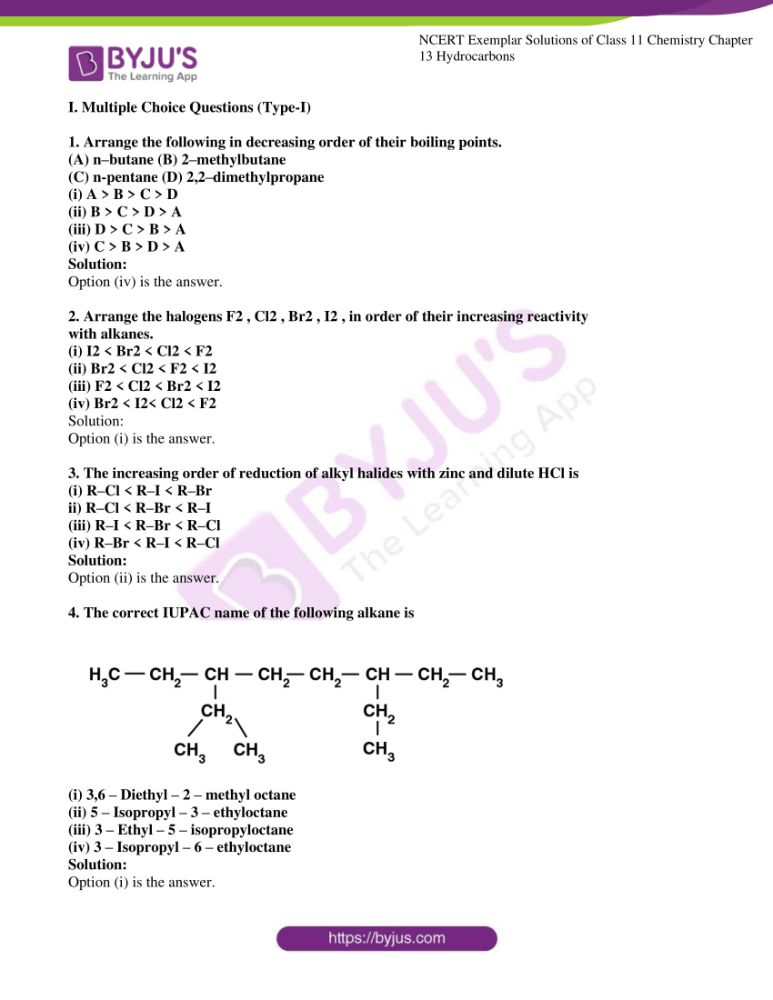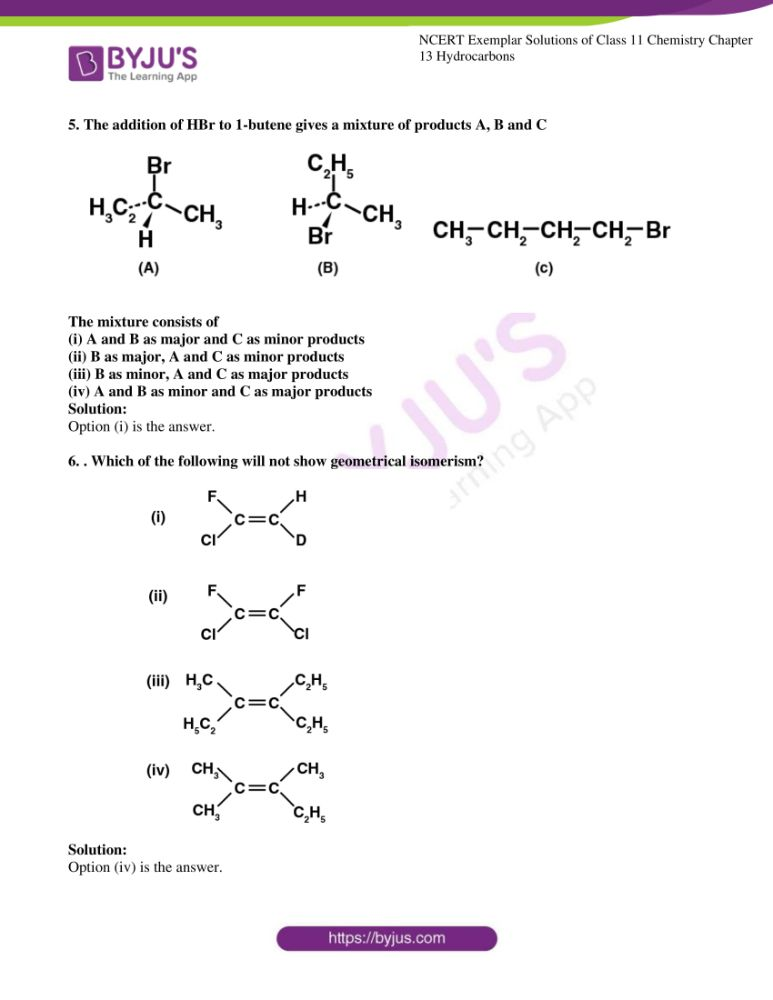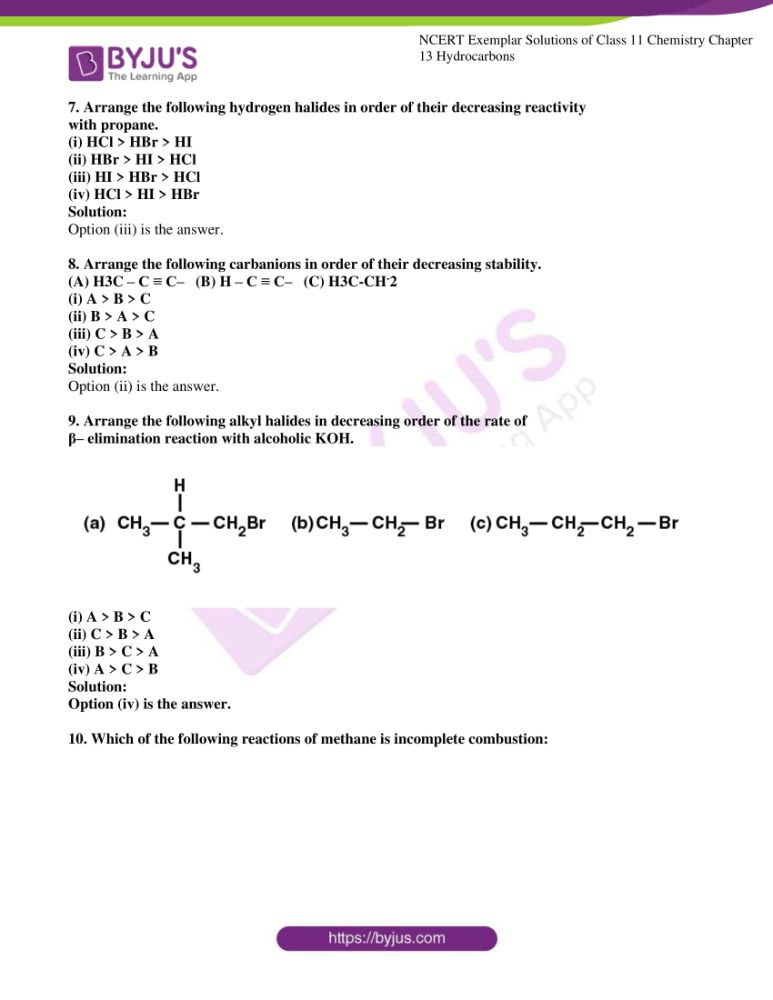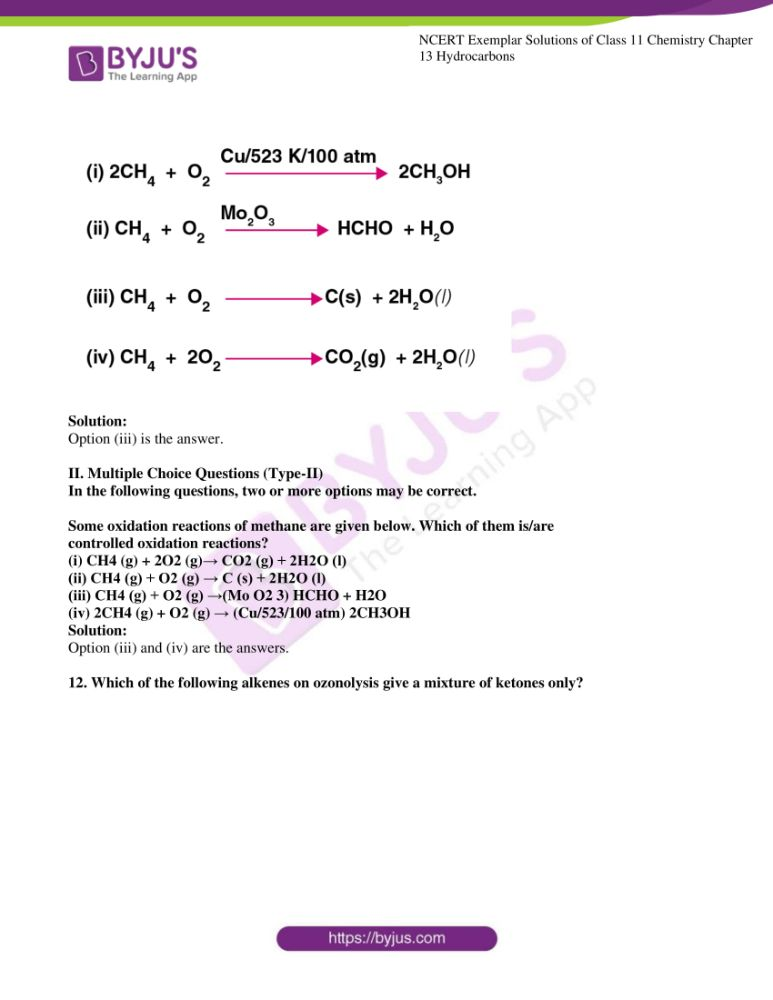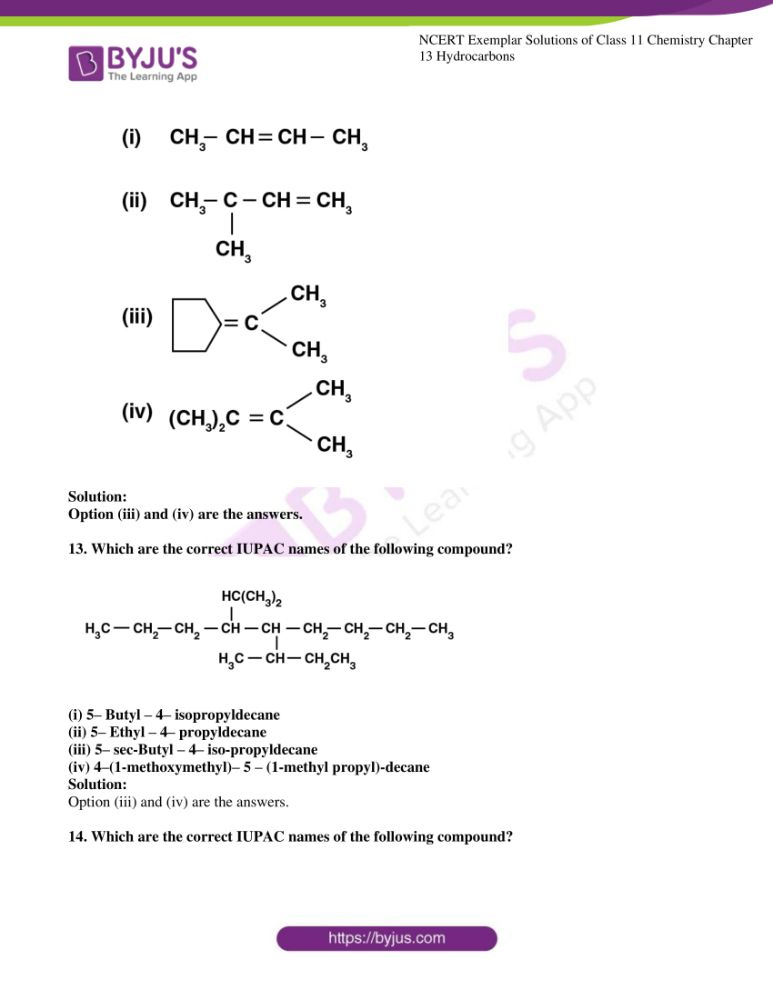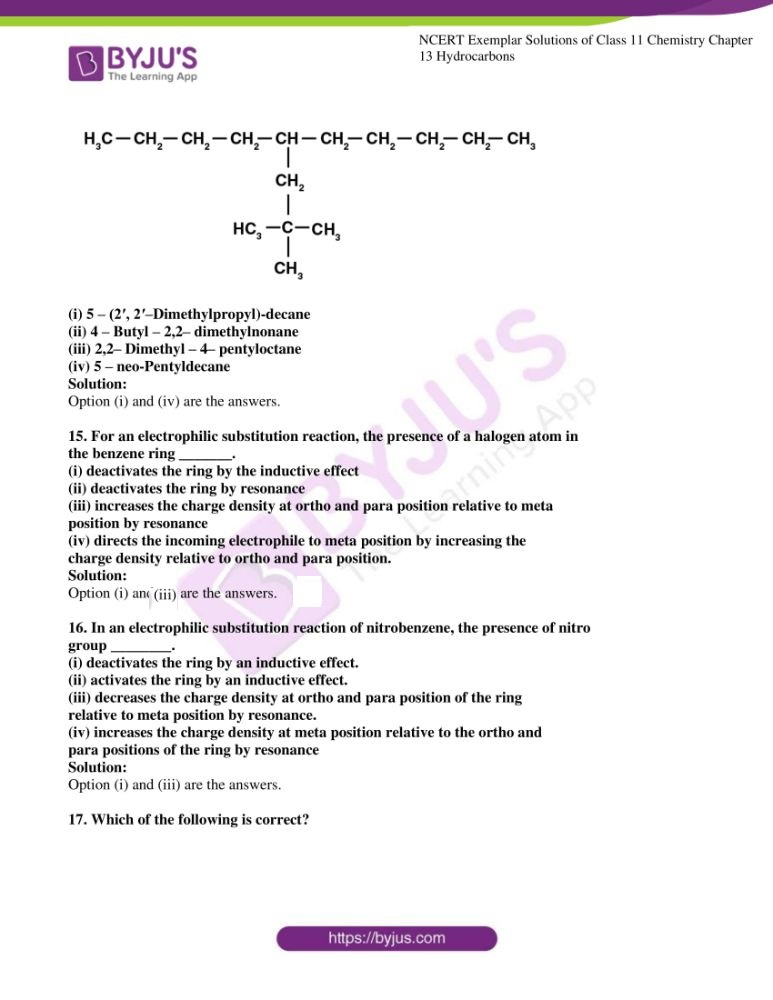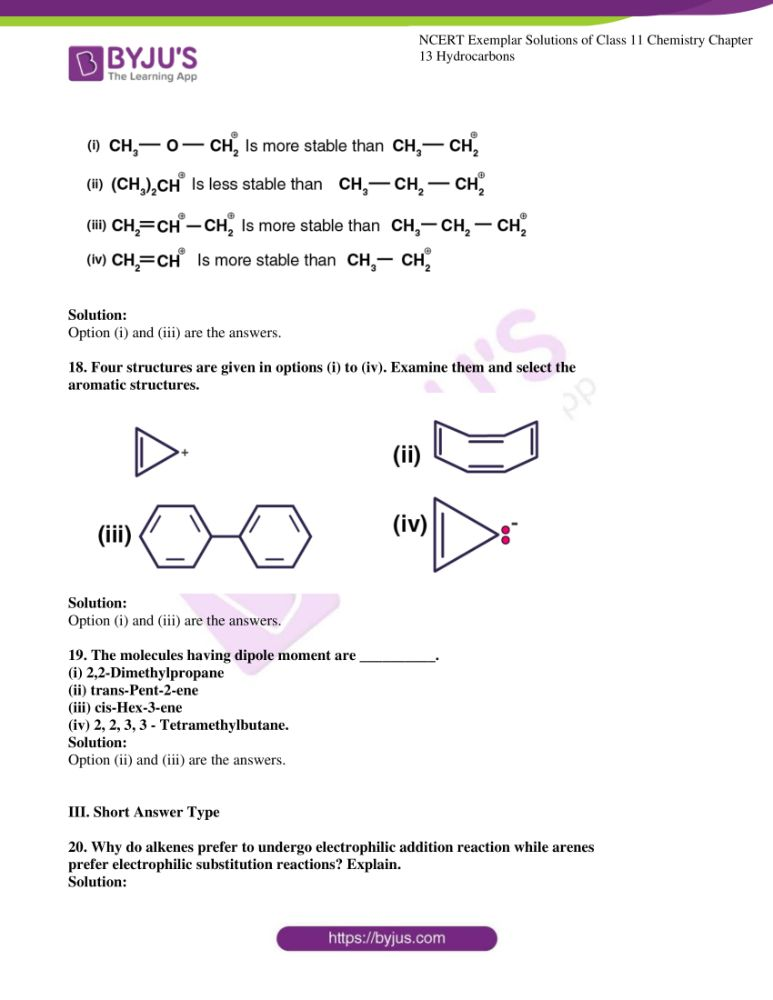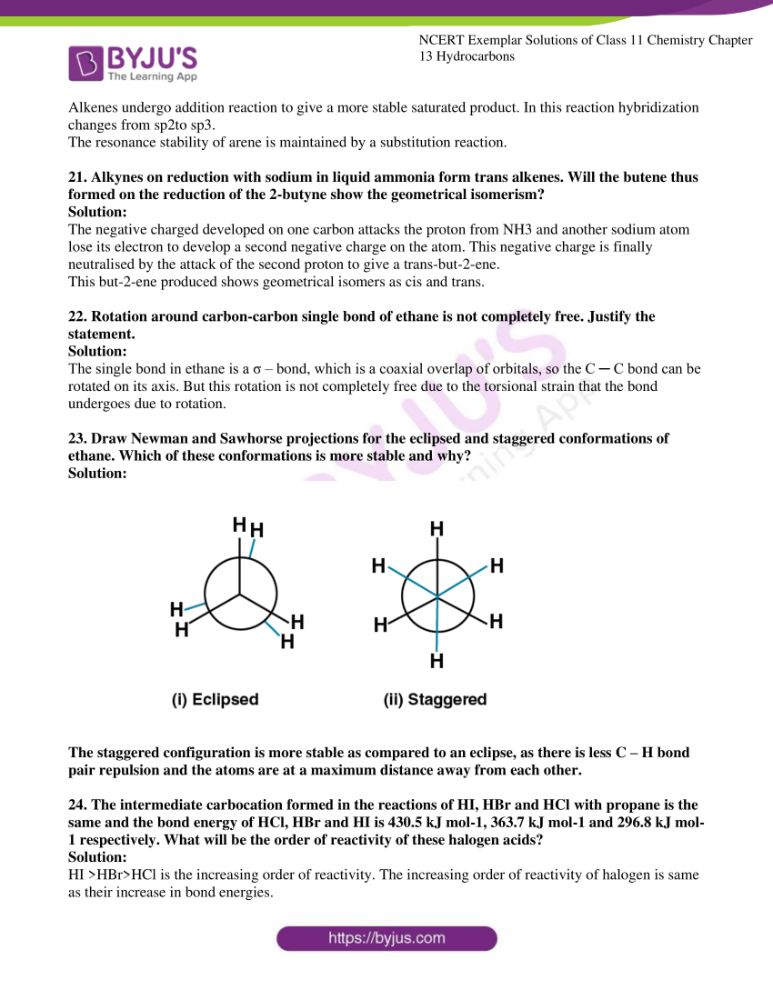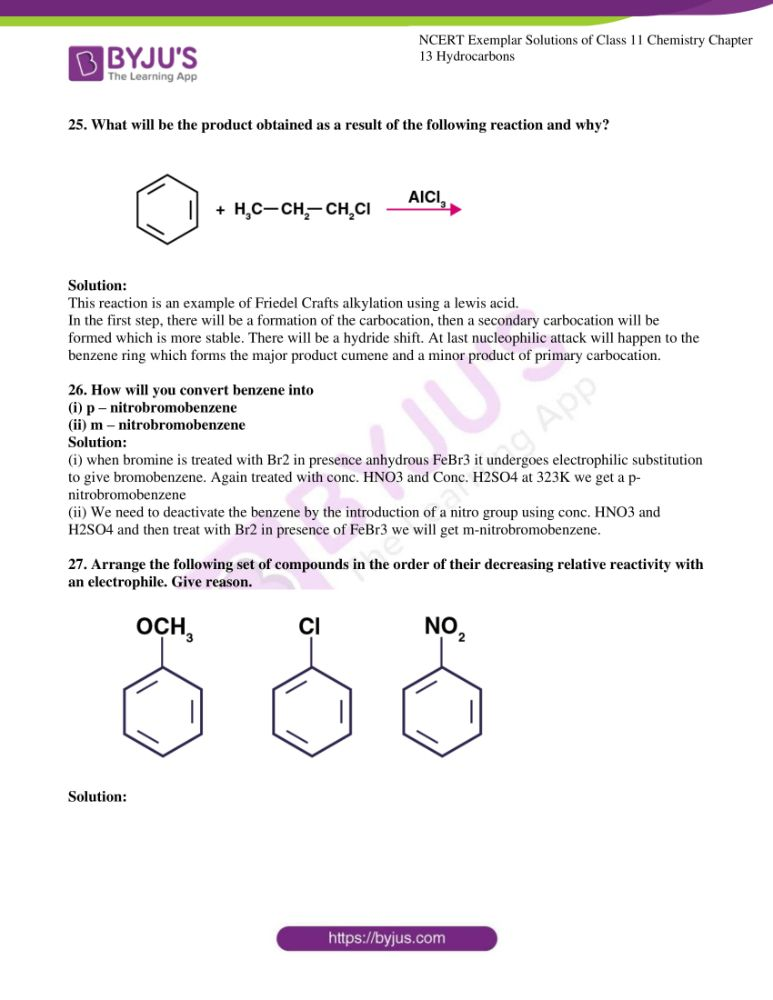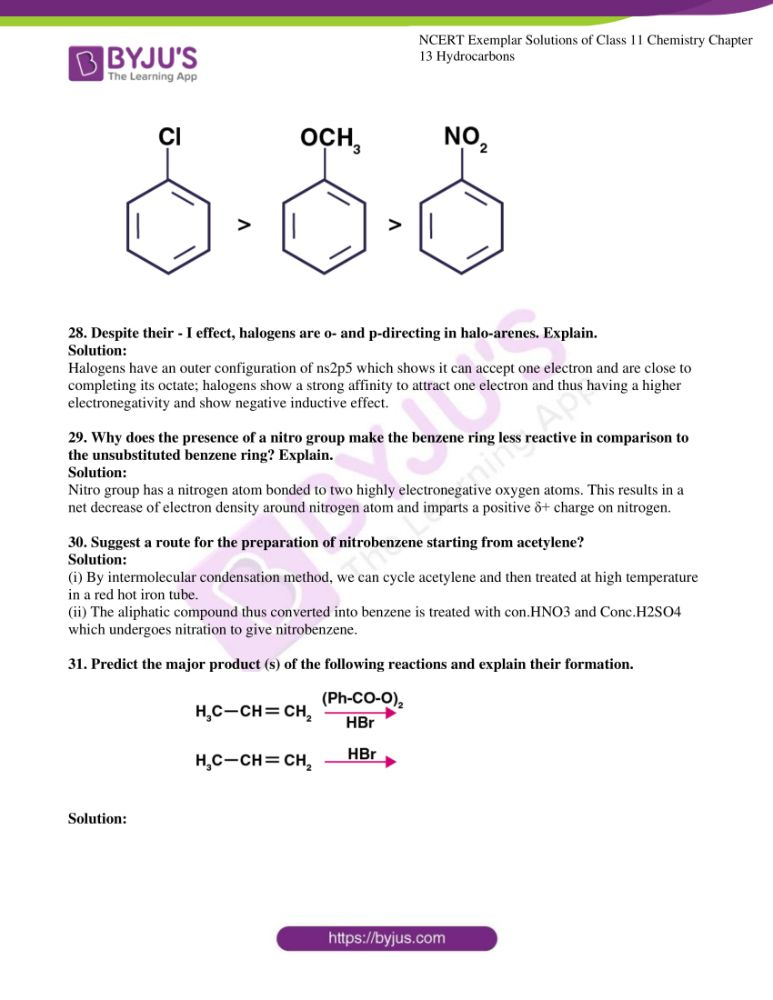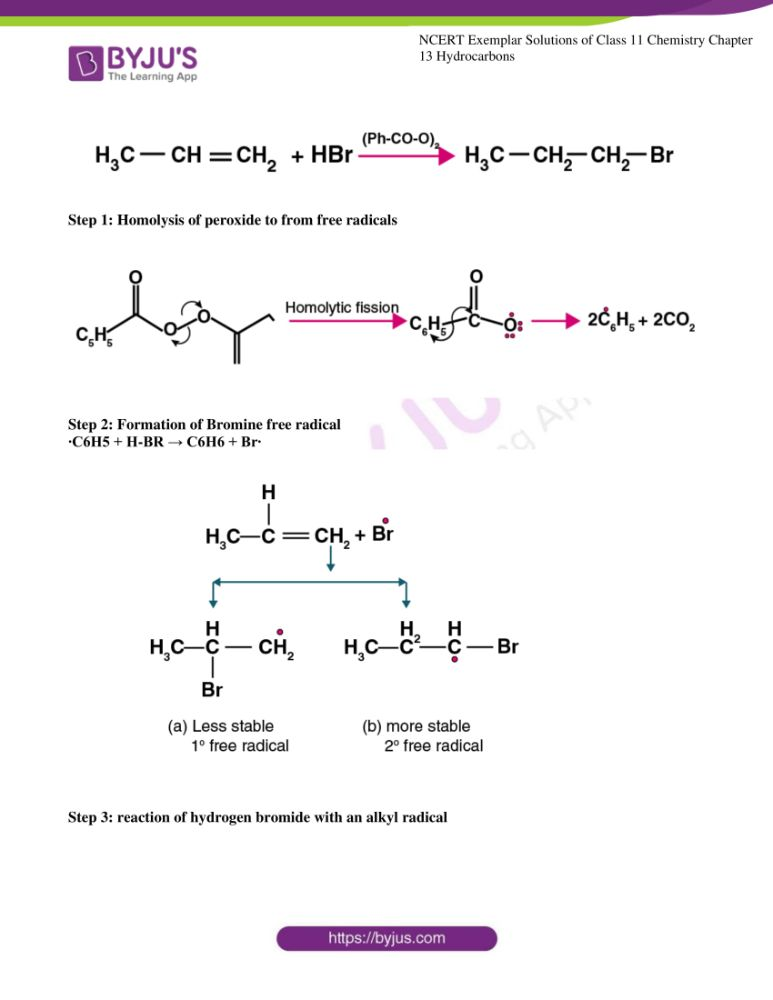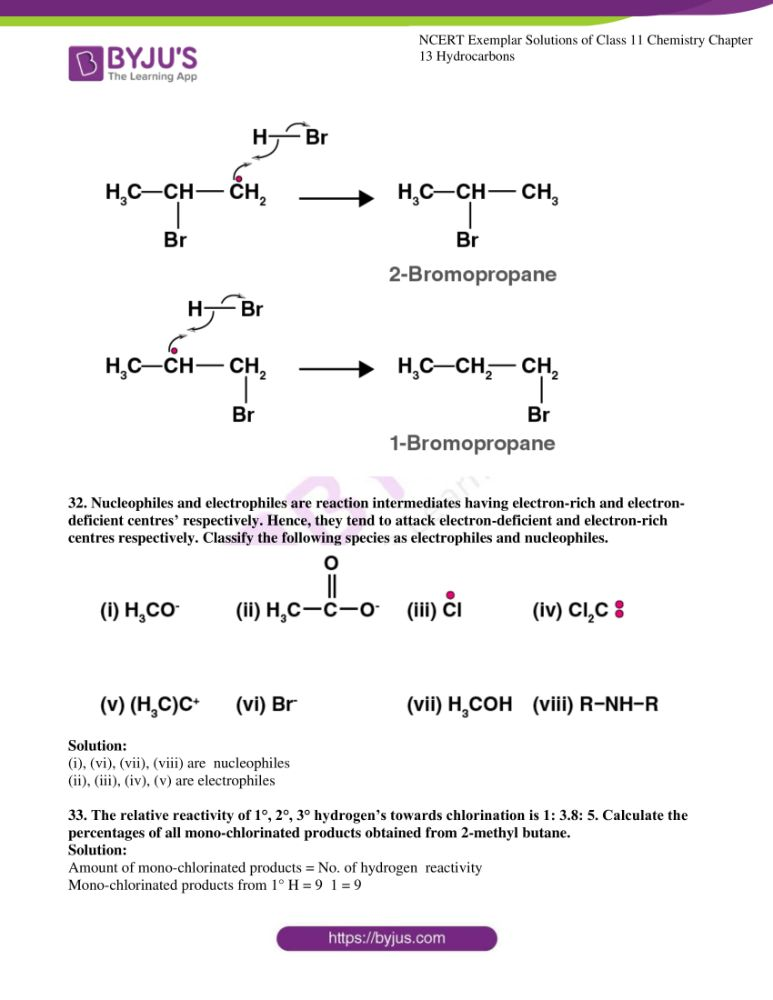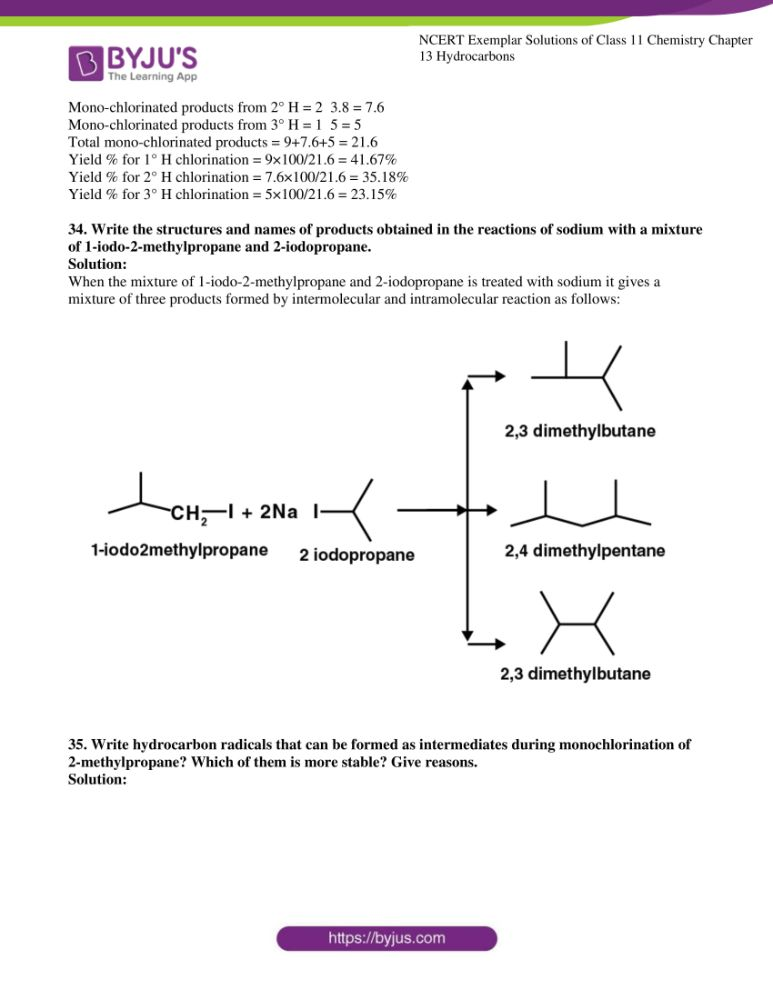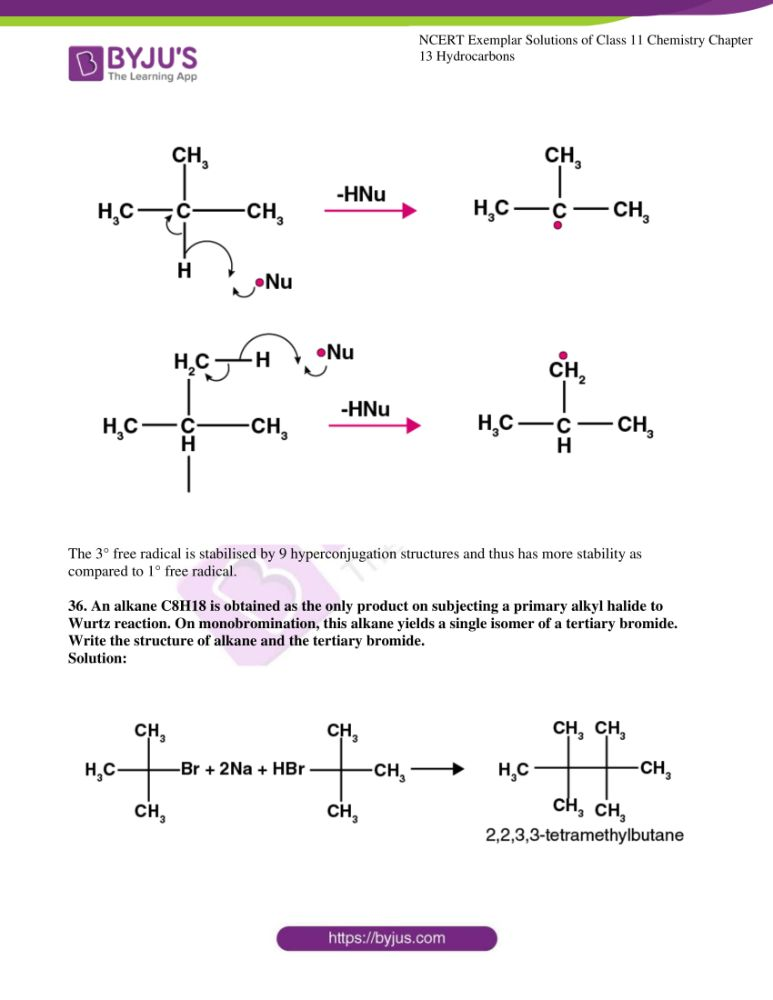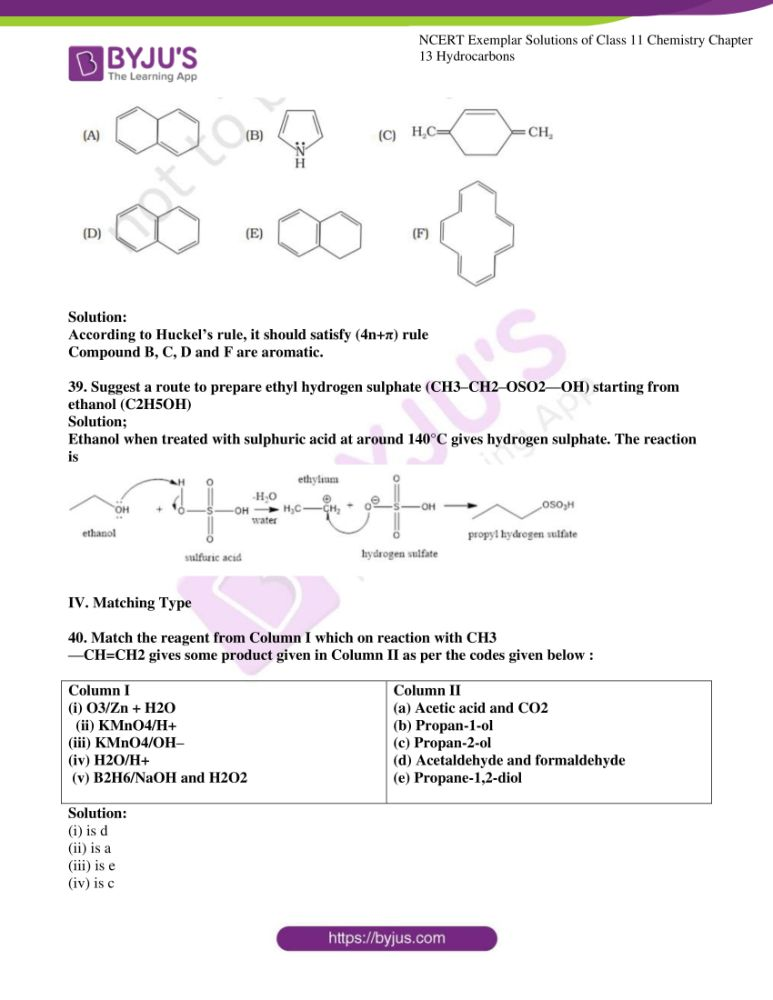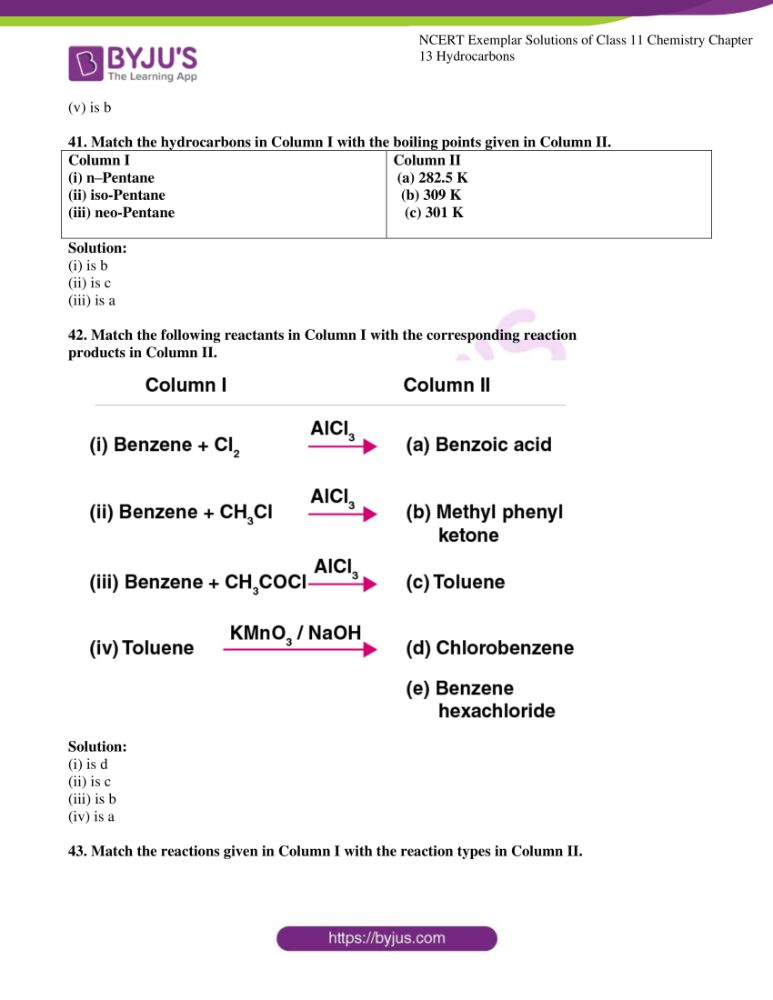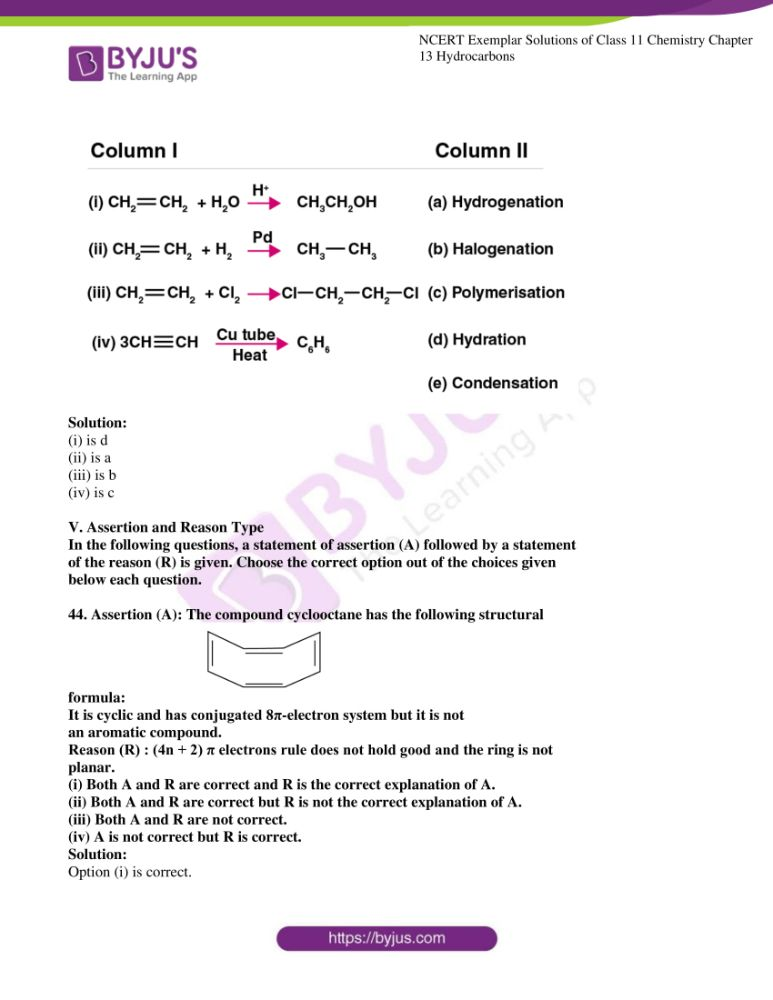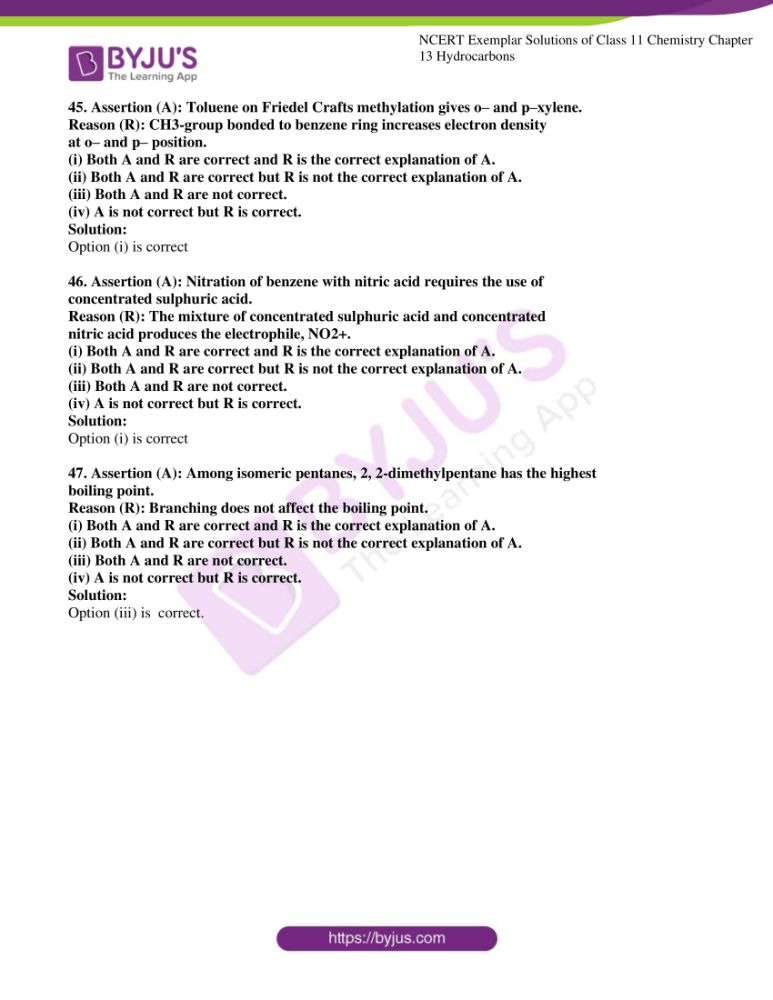### Access Answers to the NCERT Exemplar Chemistry Class 11 Chapter 13

I. Multiple Choice Questions (Type-I)

1. Arrange the following in decreasing order of their boiling points.

(A) n–butane (B) 2–methylbutane

(C) n-pentane (D) 2,2–dimethylpropane

(i) A > B > C > D

(ii) B > C > D > A

(iii) D > C > B > A

(iv) C > B > D > A

Solution:

2. Arrange the halogens F2 , Cl2 , Br2 , I2 , in order of their increasing reactivity

with alkanes.

(i) I2 < Br2 < Cl2 < F2

(ii) Br2 < Cl2 < F2 < I2

(iii) F2 < Cl2 < Br2 < I2

(iv) Br2 < I2< Cl2 < F2

Solution:

3. The increasing order of reduction of alkyl halides with zinc and dilute HCl is

(i) R–Cl < R–I < R–Br

ii) R–Cl < R–Br < R–I

(iii) R–I < R–Br < R–Cl

(iv) R–Br < R–I < R–Cl

Solution:

4. The correct IUPAC name of the following alkane is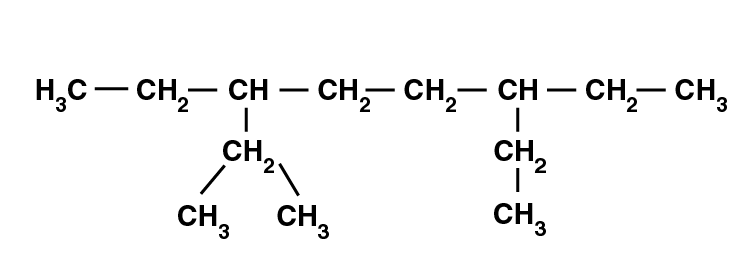(i) 3,6 – Diethyl – 2 – methyl octane

(ii) 5 – Isopropyl – 3 – ethyloctane

(iii) 3 – Ethyl – 5 – isopropyloctane

(iv) 3 – Isopropyl – 6 – ethyloctane

Solution:

5. The addition of HBr to 1-butene gives a mixture of products A, B and C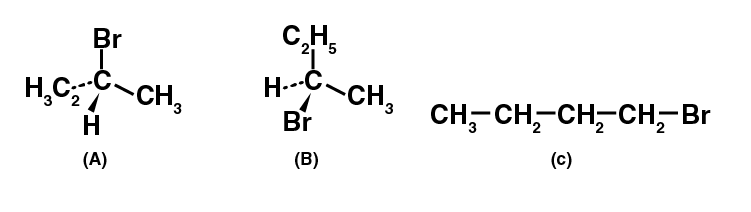The mixture consists of

(i) A and B as major and C as minor products

(ii) B as major, A and C as minor products

(iii) B as minor, A and C as major products

(iv) A and B as minor and C as major products

Solution:

6. . Which of the following will not show geometrical isomerism?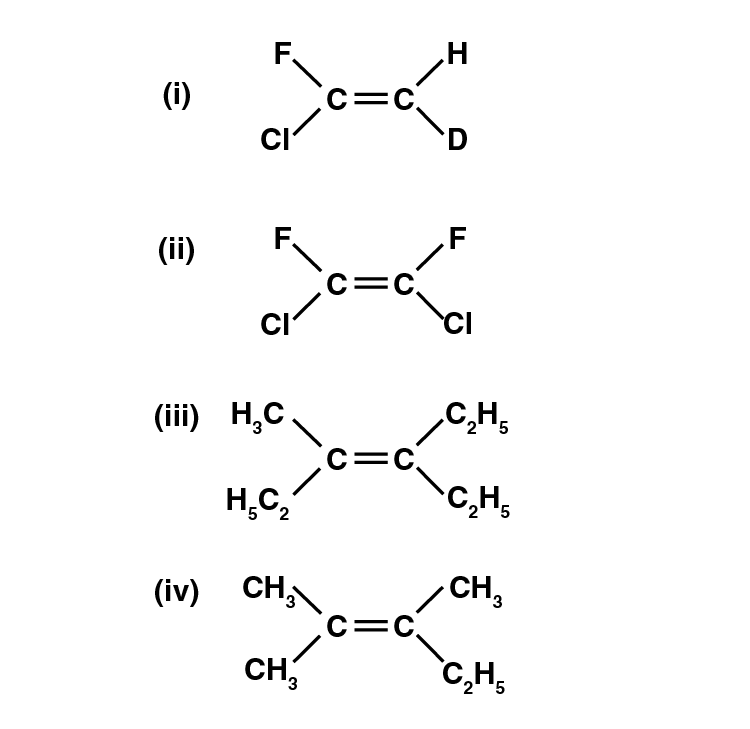Solution:

7. Arrange the following hydrogen halides in order of their decreasing reactivity

with propane.

(i) HCl > HBr > HI

(ii) HBr > HI > HCl

(iii) HI > HBr > HCl

(iv) HCl > HI > HBr

Solution:

8. Arrange the following carbanions in order of their decreasing stability.

(A) H3C – C ≡ C– (B) H – C ≡ C– (C) H3C-CH2

(i) A > B > C

(ii) B > A > C

(iii) C > B > A

(iv) C > A > B

Solution:

9. Arrange the following alkyl halides in decreasing order of the rate of

β– elimination reaction with alcoholic KOH.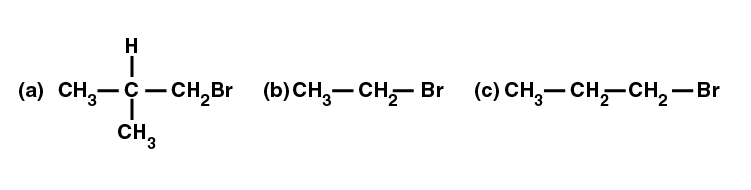(i) A > B > C

(ii) C > B > A

(iii) B > C > A

(iv) A > C > B

Solution:

10. Which of the following reactions of methane is incomplete combustion: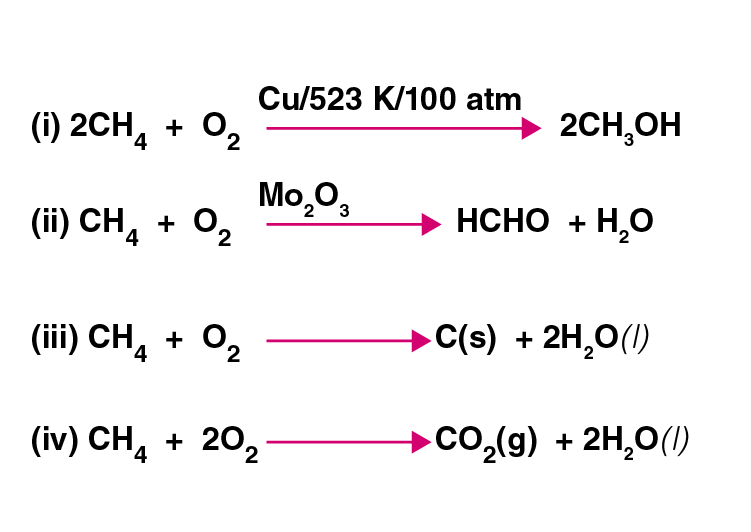Solution:

II. Multiple Choice Questions (Type-II)

In the following questions, two or more options may be correct.

Some oxidation reactions of methane are given below. Which of them is/are

controlled oxidation reactions?

(i) CH4 (g) + 2O2 (g)→ CO2 (g) + 2H2O (l)

(ii) CH4 (g) + O2 (g) → C (s) + 2H2O (l)

(iii) CH4 (g) + O2 (g) →(Mo O2 3) HCHO + H2O

(iv) 2CH4 (g) + O2 (g) → (Cu/523/100 atm) 2CH3OH

Solution:

Option (iii) and (iv) are the answers.

12. Which of the following alkenes on ozonolysis give a mixture of ketones only?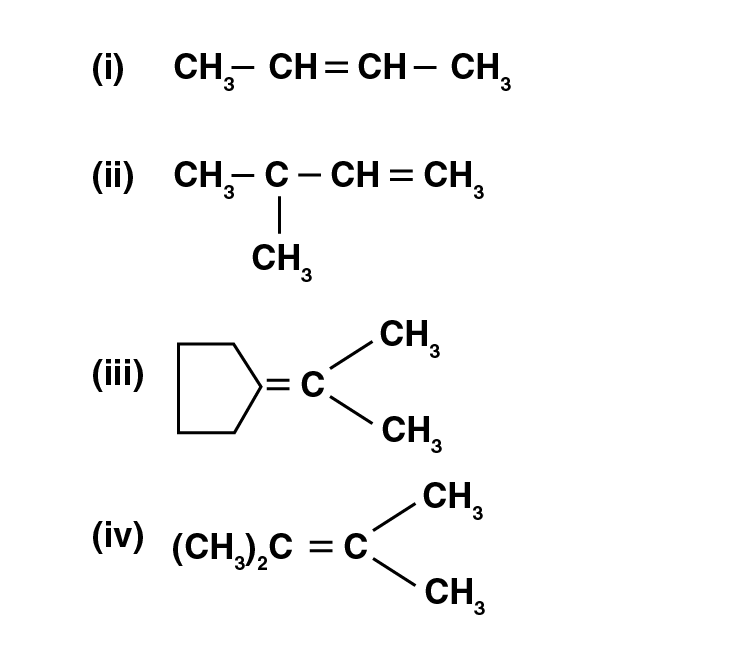Solution:

Option (iii) and (iv) are the answers.

13. Which are the correct IUPAC names of the following compound?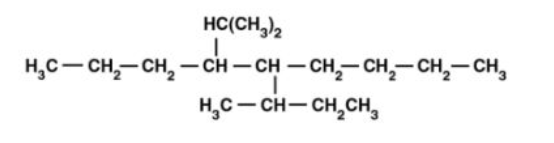(i) 5– Butyl – 4– isopropyldecane

(ii) 5– Ethyl – 4– propyldecane

(iii) 5– sec-Butyl – 4– iso-propyldecane

(iv) 4–(1-methoxymethyl)– 5 – (1-methyl propyl)-decane

Solution:

Option (iii) and (iv) are the answers.

14. Which are the correct IUPAC names of the following compound?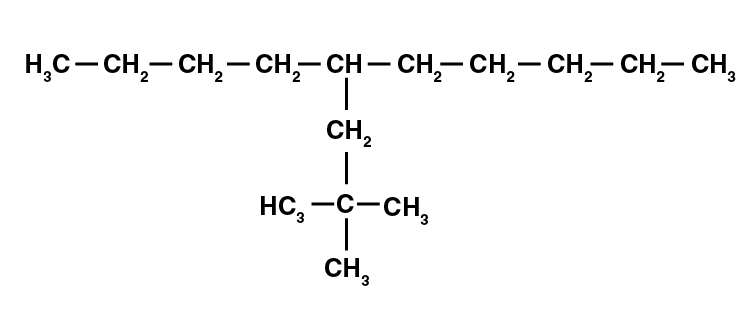(i) 5 – (2′, 2′–Dimethylpropyl)-decane

(ii) 4 – Butyl – 2,2– dimethylnonane

(iii) 2,2– Dimethyl – 4– pentyloctane

(iv) 5 – neo-Pentyldecane

Solution:

Option (i) and (iv) are the answers.

15. For an electrophilic substitution reaction, the presence of a halogen atom in

the benzene ring _______.

(i) deactivates the ring by the inductive effect

(ii) deactivates the ring by resonance

(iii) increases the charge density at ortho and para position relative to meta

position by resonance

(iv) directs the incoming electrophile to meta position by increasing the

charge density relative to ortho and para position.

Solution:

Option (i) and (iii) are the answers.

16. In an electrophilic substitution reaction of nitrobenzene, the presence of nitro

group ________.

(i) deactivates the ring by an inductive effect.

(ii) activates the ring by an inductive effect.

(iii) decreases the charge density at ortho and para position of the ring

relative to meta position by resonance.

(iv) increases the charge density at meta position relative to the ortho and

para positions of the ring by resonance

Solution:

Option (i) and (iii) are the answers.

17. Which of the following is correct?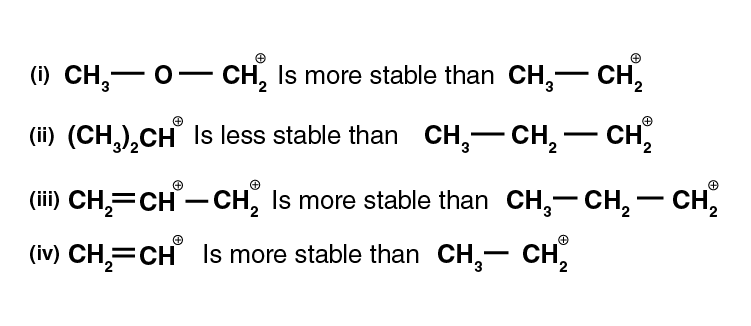Solution:

Option (i) and (iii) are the answers.

18. Four structures are given in options (i) to (iv). Examine them and select the

aromatic structures.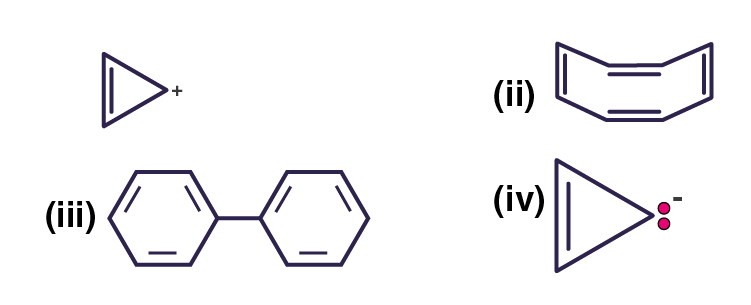Solution:

Option (i) and (iii) are the answers.

19. The molecules having dipole moment are __________.(i) 2,2-Dimethylpropane

(ii) trans-Pent-2-ene

(iii) cis-Hex-3-ene

(iv) 2, 2, 3, 3 – Tetramethylbutane.

Solution:

Option (ii) and (iii) are the answers.

20. Why do alkenes prefer to undergo electrophilic addition reaction while arenes

prefer electrophilic substitution reactions? Explain.

Solution:

Alkenes undergo addition reaction to give a more stable saturated product. In this reaction hybridization changes from sp2to sp3.

The resonance stability of arene is maintained by a substitution reaction.

21. Alkynes on reduction with sodium in liquid ammonia form trans alkenes. Will the butene thus formed on the reduction of the 2-butyne show the geometrical isomerism?

Solution:

The negative charged developed on one carbon attacks the proton from NH3 and another sodium atom lose its electron to develop a second negative charge on the atom. This negative charge is finally neutralised by the attack of the second proton to give a trans-but-2-ene.

This but-2-ene produced shows geometrical isomers as cis and trans.

22. Rotation around carbon-carbon single bond of ethane is not completely free. Justify the statement.

Solution:

The single bond in ethane is a σ – bond, which is a coaxial overlap of orbitals, so the C ─ C bond can be rotated on its axis. But this rotation is not completely free due to the torsional strain that the bond undergoes due to rotation.

23. Draw Newman and Sawhorse projections for the eclipsed and staggered conformations of ethane. Which of these conformations is more stable and why?

Solution: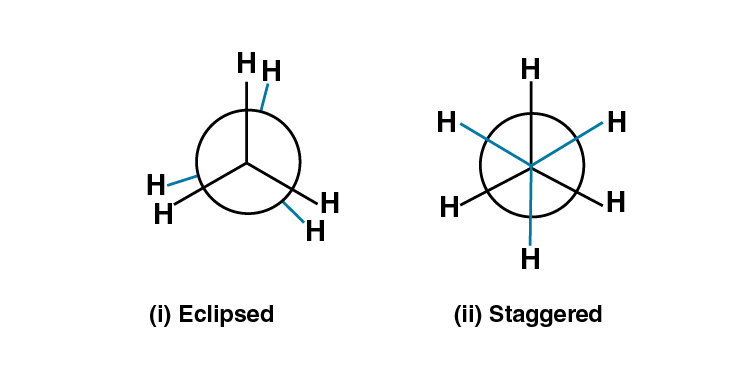The staggered configuration is more stable as compared to an eclipse, as there is less C – H bond pair repulsion and the atoms are at a maximum distance away from each other.

24. The intermediate carbocation formed in the reactions of HI, HBr and HCl with propane is the same and the bond energy of HCl, HBr and HI is 430.5 kJ mol-1, 363.7 kJ mol-1 and 296.8 kJ mol-1 respectively. What will be the order of reactivity of these halogen acids?

Solution:

HI >HBr>HCl is the increasing order of reactivity. The increasing order of reactivity of halogen is same as their increase in bond energies.

25. What will be the product obtained as a result of the following reaction and why?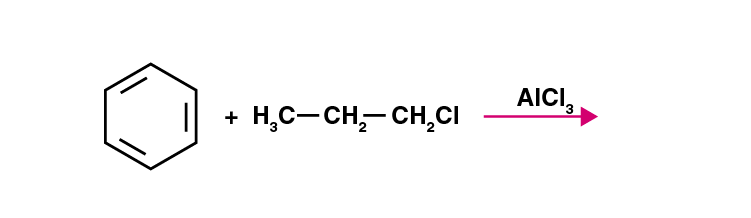Solution:

This reaction is an example of Friedel Crafts alkylation using a lewis acid.

In the first step, there will be a formation of the carbocation, then a secondary carbocation will be formed which is more stable. There will be a hydride shift. At last nucleophilic attack will happen to the benzene ring which forms the major product cumene and a minor product of primary carbocation.

26. How will you convert benzene into

(i) p – nitrobromobenzene

(ii) m – nitrobromobenzene

Solution:

(i) when bromine is treated with Br2 in presence anhydrous FeBr3 it undergoes electrophilic substitution to give bromobenzene. Again treated with conc. HNO3 and Conc. H2SO4 at 323K we get a p-nitrobromobenzene

(ii) We need to deactivate the benzene by the introduction of a nitro group using conc. HNO3 and H2SO4 and then treat with Br2 in presence of FeBr3 we will get m-nitrobromobenzene.

27. Arrange the following set of compounds in the order of their decreasing relative reactivity with an electrophile. Give reason.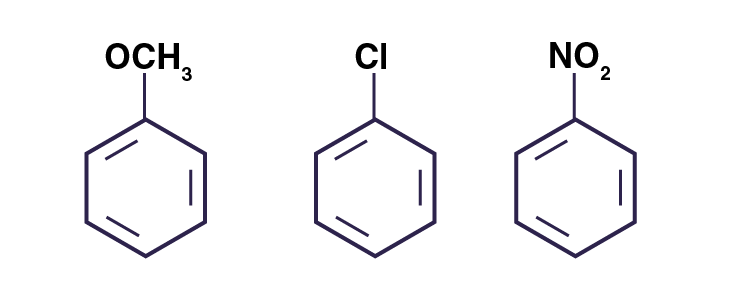Solution: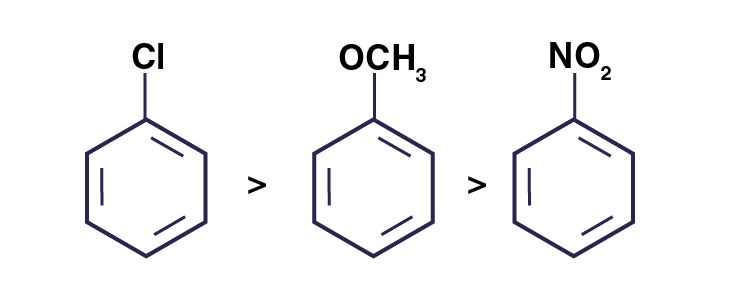28. Despite their – I effect, halogens are o- and p-directing in halo-arenes. Explain.

Solution:

Halogens have an outer configuration of ns2p5 which shows it can accept one electron and are close to completing its octate; halogens show a strong affinity to attract one electron and thus having a higher electronegativity and show negative inductive effect.

29. Why does the presence of a nitro group make the benzene ring less reactive in comparison to the unsubstituted benzene ring? Explain.

Solution:

Nitro group has a nitrogen atom bonded to two highly electronegative oxygen atoms. This results in a net decrease of electron density around nitrogen atom and imparts a positive δ+ charge on nitrogen.

30. Suggest a route for the preparation of nitrobenzene starting from acetylene?

Solution:

(i) By intermolecular condensation method, we can cycle acetylene and then treated at high temperature in a red hot iron tube.

(ii) The aliphatic compound thus converted into benzene is treated with con.HNO3 and Conc.H2SO4 which undergoes nitration to give nitrobenzene.

31. Predict the major product (s) of the following reactions and explain their formation.Solution: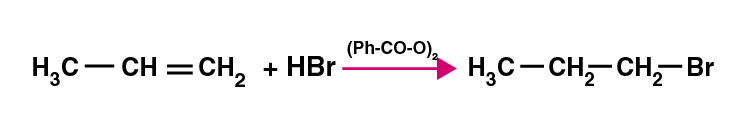Step 1: Homolysis of peroxide to from free radicals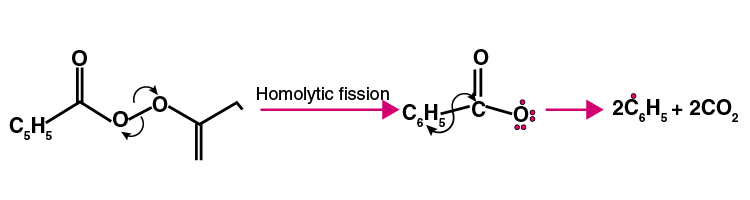Step 2: Formation of Bromine free radical

∙C6H5 + H-BR → C6H6 + Br∙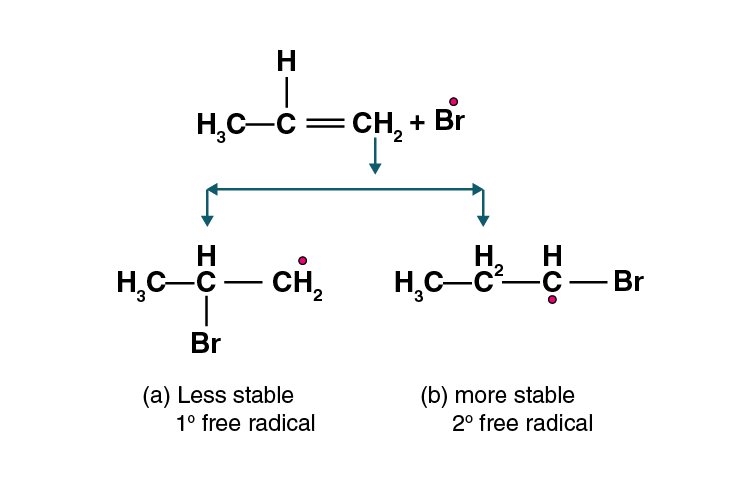Step 3: reaction of hydrogen bromide with an alkyl radical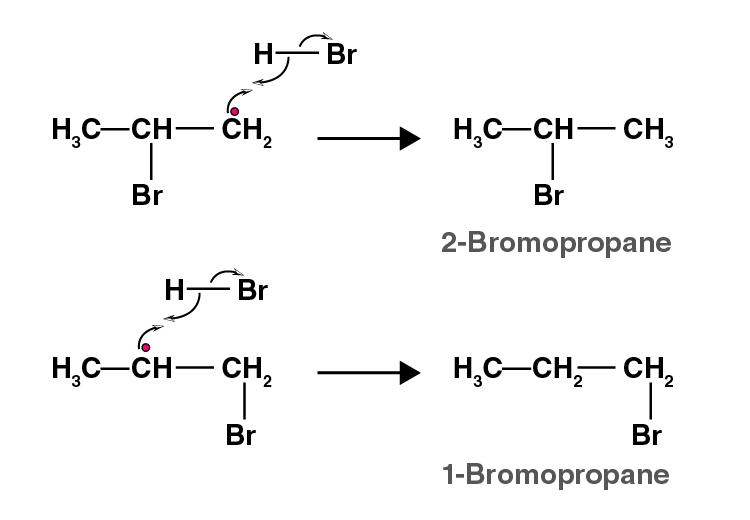32. Nucleophiles and electrophiles are reaction intermediates having electron-rich and electron-deficient centres’ respectively. Hence, they tend to attack electron-deficient and electron-rich centres respectively. Classify the following species as electrophiles and nucleophiles.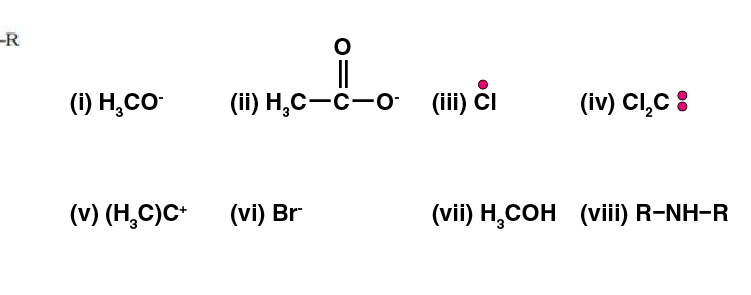Solution:

(i), (vi), (vii), (viii) are nucleophiles

(ii), (iii), (iv), (v) are electrophiles

33. The relative reactivity of 1°, 2°, 3° hydrogen’s towards chlorination is 1: 3.8: 5. Calculate the percentages of all mono-chlorinated products obtained from 2-methyl butane.

Solution:

Amount of mono-chlorinated products = No. of hydrogen reactivity

Mono-chlorinated products from 1° H = 9 1 = 9

Mono-chlorinated products from 2° H = 2 3.8 = 7.6

Mono-chlorinated products from 3° H = 1 5 = 5

Total mono-chlorinated products = 9+7.6+5 = 21.6

Yield % for 1° H chlorination = 9×100/21.6 = 41.67%

Yield % for 2° H chlorination = 7.6×100/21.6 = 35.18%

Yield % for 3° H chlorination = 5×100/21.6 = 23.15%

34. Write the structures and names of products obtained in the reactions of sodium with a mixture of 1-iodo-2-methylpropane and 2-iodopropane.

Solution:

When the mixture of 1-iodo-2-methylpropane and 2-iodopropane is treated with sodium it gives a mixture of three products formed by intermolecular and intramolecular reaction as follows: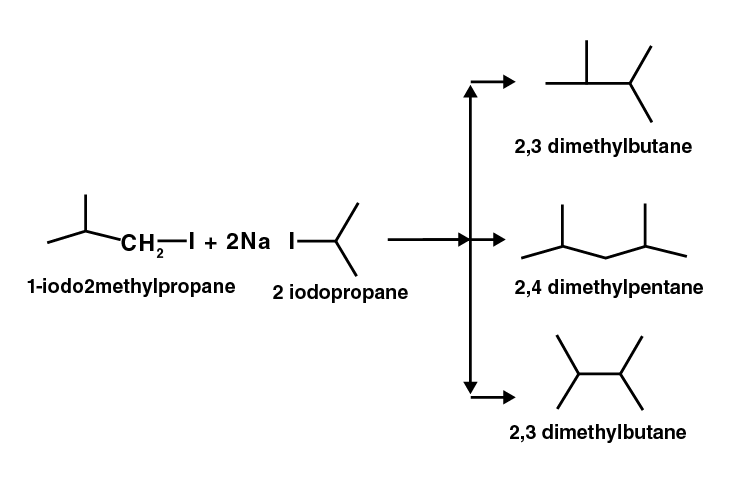35. Write hydrocarbon radicals that can be formed as intermediates during monochlorination of 2-methylpropane? Which of them is more stable? Give reasons.

Solution: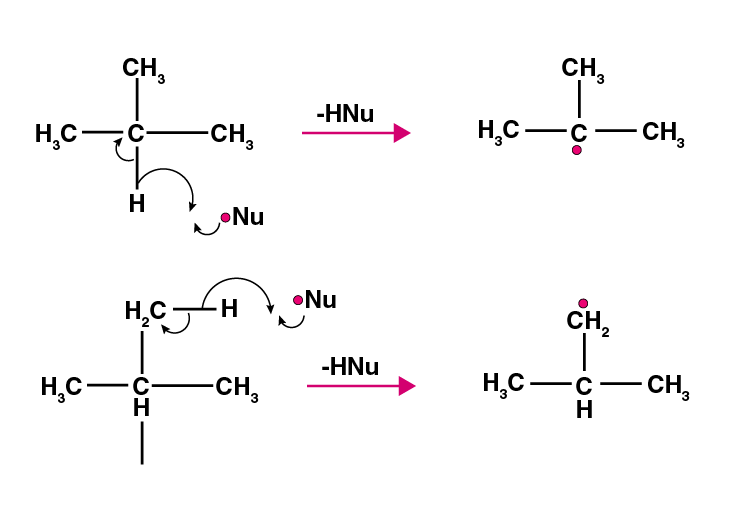The 3° free radical is stabilised by 9 hyperconjugation structures and thus has more stability as compared to 1° free radical.

36. An alkane C8H18 is obtained as the only product on subjecting a primary alkyl halide to Wurtz reaction. On monobromination, this alkane yields a single isomer of a tertiary bromide. Write the structure of alkane and the tertiary bromide.

Solution: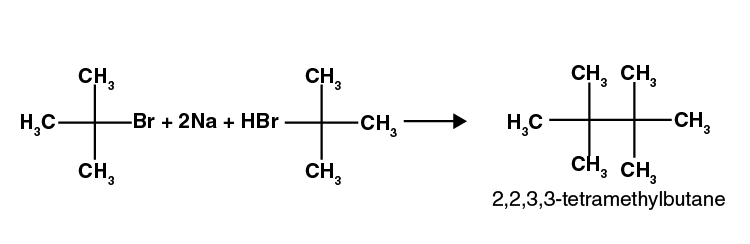37. The ring systems having the following characteristics are aromatic.

(i) Planar ring containing conjugated π bonds.

(ii) Complete delocalisation of the π−electrons in-ring system i.e. each atom in the ring has unhybridised p-orbital, and

(iii) Presence of (4n+2)−electrons in the ring where n is an integer (n = 0, 1, 2,………..) [Huckel rule].

Using this information classifies the following compounds as aromatic/non-aromatic.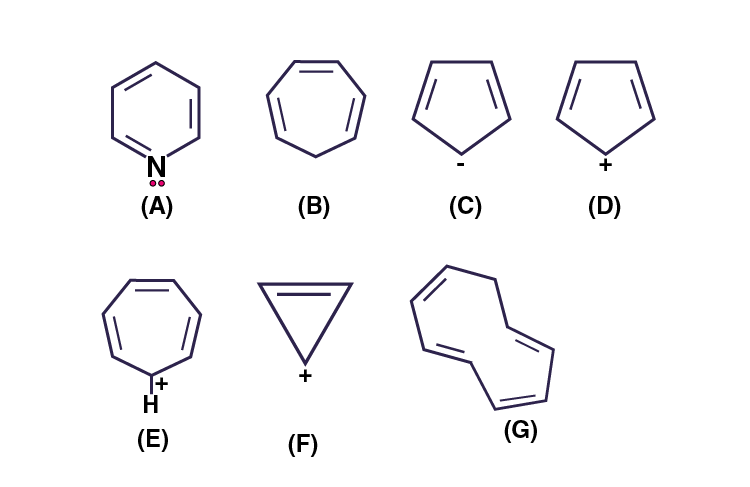Solution:

Aromatic compounds: A, E and F

Non-Aromatic : B, C, D and G

38. Which of the following compounds are aromatic according to Huckel’s rule?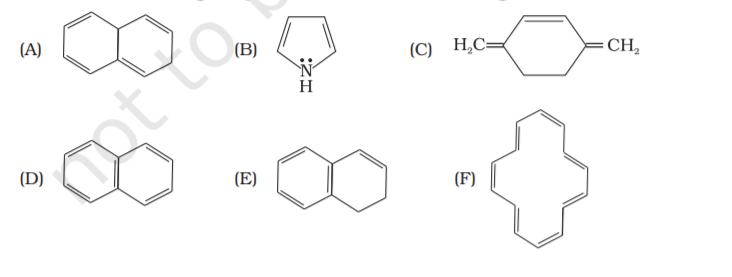Solution:

According to Huckel’s rule, it should satisfy (4n+π) rule

Compound B, C, D and F are aromatic.

39. Suggest a route to prepare ethyl hydrogen sulphate (CH3–CH2–OSO2—OH) starting from ethanol (C2H5OH)

Solution;

Ethanol when treated with sulphuric acid at around 140°C gives hydrogen sulphate. The reaction is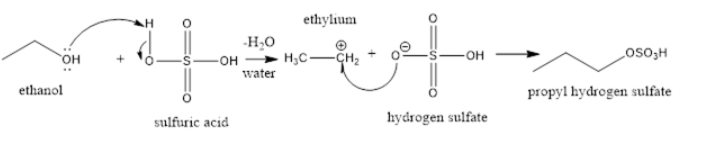IV. Matching Type

40. Match the reagent from Column I which on reaction with CH3

—CH=CH2 gives some product given in Column II as per the codes given below :

 Column I (i) O3/Zn + H2O (ii) KMnO4/H+ (iii) KMnO4/OH– (iv) H2O/H+ (v) B2H6/NaOH and H2O2 Column II (a) Acetic acid and CO2 (b) Propan-1-ol (c) Propan-2-ol (d) Acetaldehyde and formaldehyde (e) Propane-1,2-diol

Solution:

(i) is d

(ii) is a

(iii) is e

(iv) is c

(v) is b

41. Match the hydrocarbons in Column I with the boiling points given in Column II.

 Column I (i) n–Pentane (ii) iso-Pentane (iii) neo-Pentane Column II (a) 282.5 K (b) 309 K (c) 301 K

Solution:

(i) is b

(ii) is c

(iii) is a

42. Match the following reactants in Column I with the corresponding reaction

products in Column II.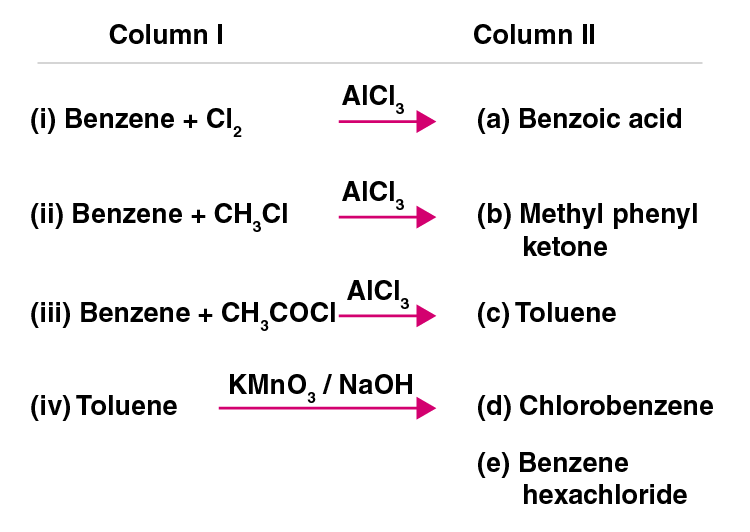Solution:

(i) is d

(ii) is c

(iii) is b

(iv) is a

43. Match the reactions given in Column I with the reaction types in Column II.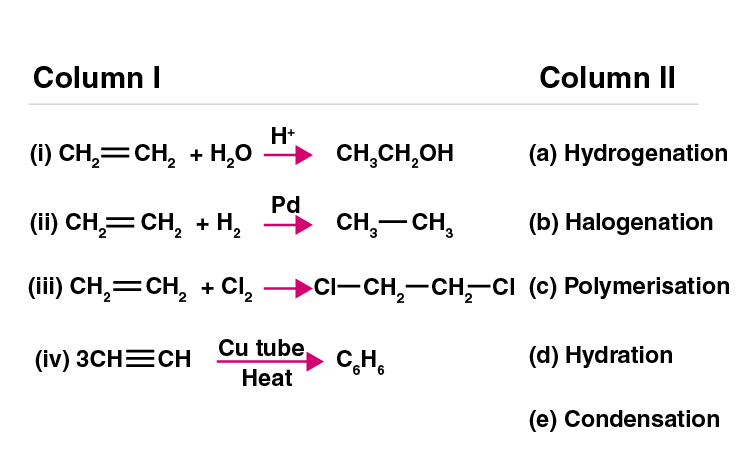Solution:

(i) is d

(ii) is a

(iii) is b

(iv) is c

V. Assertion and Reason Type

In the following questions, a statement of assertion (A) followed by a statement

of the reason (R) is given. Choose the correct option out of the choices given

below each question.

44. Assertion (A): The compound cyclooctane has the following structural

formula:It is cyclic and has conjugated 8π-electron system but it is not

an aromatic compound.

Reason (R) : (4n + 2) π electrons rule does not hold good and the ring is not

planar.

(i) Both A and R are correct and R is the correct explanation of A.

(ii) Both A and R are correct but R is not the correct explanation of A.

(iii) Both A and R are not correct.

(iv) A is not correct but R is correct.

Solution:

Option (i) is correct.

45. Assertion (A): Toluene on Friedel Crafts methylation gives o– and p–xylene.

Reason (R): CH3-group bonded to benzene ring increases electron density

at o– and p– position.

(i) Both A and R are correct and R is the correct explanation of A.

(ii) Both A and R are correct but R is not the correct explanation of A.

(iii) Both A and R are not correct.

(iv) A is not correct but R is correct.

Solution:

Option (i) is correct

46. Assertion (A): Nitration of benzene with nitric acid requires the use of

concentrated sulphuric acid.

Reason (R): The mixture of concentrated sulphuric acid and concentrated

nitric acid produces the electrophile, NO2+.

(i) Both A and R are correct and R is the correct explanation of A.

(ii) Both A and R are correct but R is not the correct explanation of A.

(iii) Both A and R are not correct.

(iv) A is not correct but R is correct.

Solution:

Option (i) is correct

47. Assertion (A): Among isomeric pentanes, 2, 2-dimethylpentane has the highest

boiling point.

Reason (R): Branching does not affect the boiling point.

(i) Both A and R are correct and R is the correct explanation of A.

(ii) Both A and R are correct but R is not the correct explanation of A.

(iii) Both A and R are not correct.

(iv) A is not correct but R is correct.

Solution:

Option (iii) is correct.

Solve the NCERT Exemplar Class 11 Chemistry Chapter 13 Hydrocarbons to understand the chapter in an easy way.

#### Important Topics of Class 11 Chapter 13 Chemistry Hydrocarbons

1. Classification
2. Alkanes
1. Nomenclature and Isomerism
2. Preparation
3. Properties
4. Conformations
3. Alkenes
1. Structure of Double Bond
2. Nomenclature
3. Isomerism
4. Preparation
5. Properties
4. Alkynes
1. Nomenclature and Isomerism
2. Structure of Triple Bond
3. Preparation
4. Properties
5. Aromatic Hydrocarbon
1. Nomenclature and Isomerism
2. Structure of Benzene
3. Aromaticity
4. Preparation of Benzene
5. Properties
6. Directive Influence of a Functional Group in Monosubstituted Benzene
6. Carcinogenicity and Toxicity

Hydrocarbons lay a foundation for advanced topics, which you will be learning in the higher classes. If you are planning to take a science- or medical-related course, it is important to get good knowledge on this topic to avoid difficulty in future. For study materials of NCERT Class 11 and Class 12 Science and a comprehensive learning experience, visit BYJU’S website or download BYJU’S – The Learning App.

## Frequently Asked Questions on NCERT Exemplar Solutions for Class 11 Chemistry Chapter 13

Q1

### What are the concepts discussed in Chapter 13 of NCERT Exemplar Solutions for Class 11 Chemistry?

Important topics of Class 11 Chapter 13 Chemistry Hydrocarbons are
1. Classification
2. Alkanes – Nomenclature and Isomerism, Preparation, Properties and Conformations
3. Alkenes – Structure of Double Bond, Nomenclature, Isomerism, Preparation and Properties
4. Alkynes – Nomenclature and Isomerism, Structure of Triple Bond, Preparation and Properties
5. Aromatic Hydrocarbon – Nomenclature and Isomerism, Structure of Benzene, Aromaticity, Preparation of Benzene, Properties, and Directive Influence of a Functional Group in Monosubstituted Benzene
6. Carcinogenicity and Toxicity
Q2

### How should I prepare Chapter 13 of NCERT Exemplar Solutions for Class 11 Chemistry for the annual exam?

The classification of hydrocarbons is an important topic for the annual exam and other competitive exams. Students should understand the nomenclature and isomerism, preparation, properties, and conformations of alkanes, alkenes and alkynes while preparing this chapter. Carcinogenicity and toxicity should be learnt in detail, along with the reaction. Studying these topics using the NCERT Exemplar Solutions from BYJU’S will provide proper guidance about the concepts which are important from the exam point of view.
Q3

### How is BYJU’S NCERT Exemplar Solutions for Class 11 Chemistry Chapter 13 PDF useful for students?

The concept of alkanes, alkenes and alkynes requires conceptual clarity among students. In order to perform well in the final exam, students should possess comprehensive knowledge about the topics present in each chapter. While solving questions from the prescribed textbook, students can opt for a perfect study material which satisfies all their needs. The PDF format of solutions is available on BYJU’S, which can be downloaded by students to get their doubts clarified instantly.